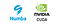# 3D Cuda Kernel in NumbaCourtesy: https://medium.com/rapids-ai/the-life-of-a-numba-kernel-a-compilation-pipeline-taking-user-defined-functions-in-python-to-cuda-71cc39b77625

There aren’t enough tutorials on writing 3D Cuda kernel in Numba so I decided to share my learnings here. In this small example, we will write a Cuda kernel (calculate_mean) to calculate the mean value of neighboring voxels for each voxel in a 3D volume.

There are few things we need to keep in mind while launching a kernel for example there are certain limits on the maximum number of Cuda blocks and the maximum number of threads inside each block. Taking this into consideration we calculate a feasible set of block and thread dimensions.

Here, the dimension of volume…